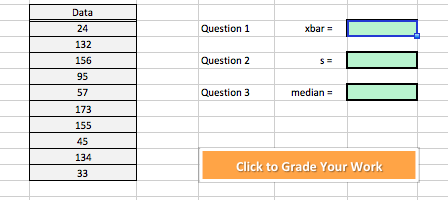Data24132156955717315545134Question1xbarQuestion 2Question 3 medanmedianClick to Grade Your Work

Questionhelp_outlineImage TranscriptioncloseData 24 132 156 95 57 173 155 45 134 Question1 xbar Question 2 Question 3 medan median Click to Grade Your Work fullscreen
Step 1

The xbar for the given data in the question represents the mean of the data.

The formula to calculate the mean of the question is given as;

In the given question,  n = 10

Step 2

And s represents the standard deviation for the given data.

The formula to calculate s is given as;

Step 3

Now, to calculate the mean and standard deviation for the given d...

Want to see the full answer?

See Solution

Want to see this answer and more?

Our solutions are written by experts, many with advanced degrees, and available 24/7

See Solution
Tagged in

Statistics